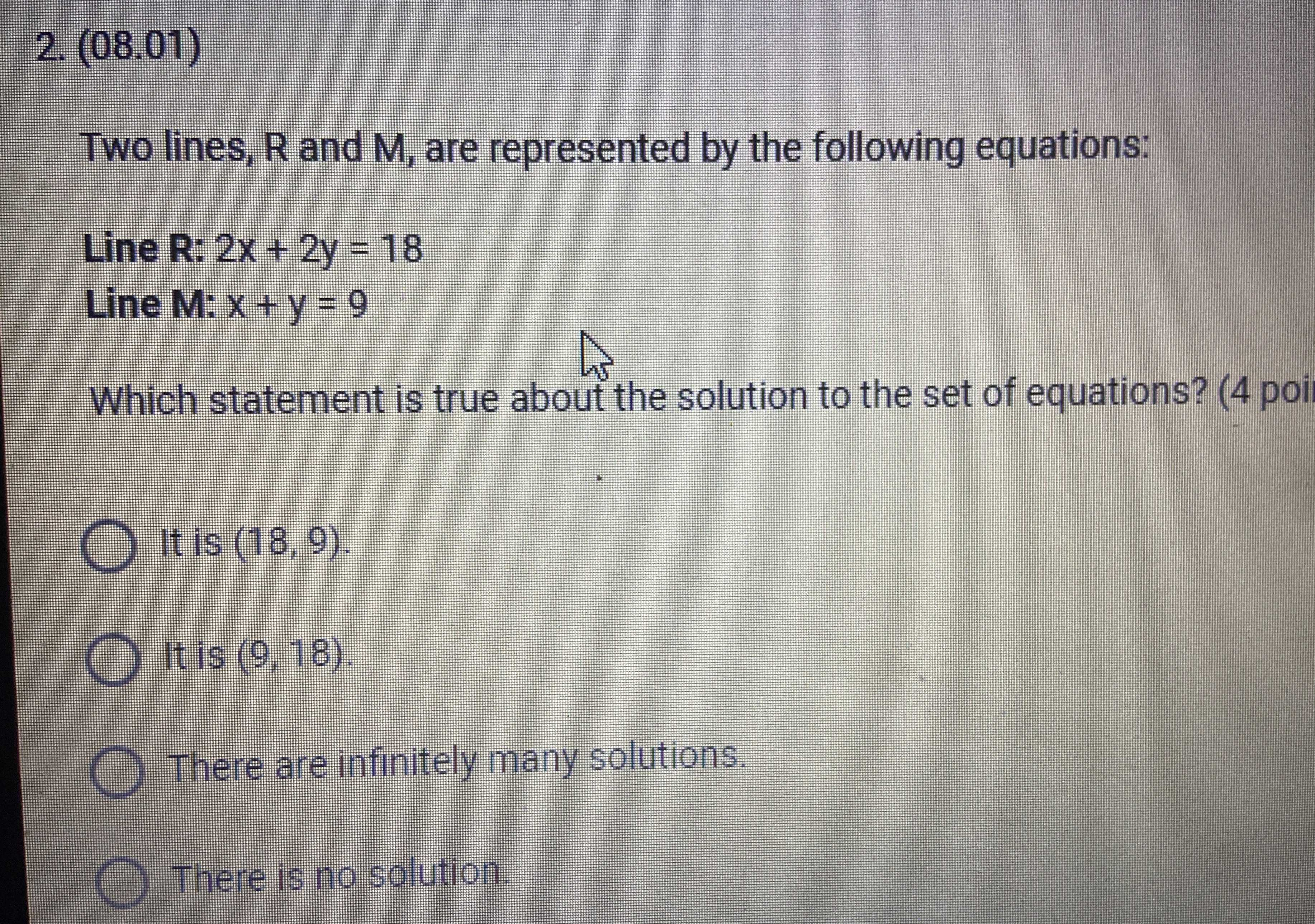### ¿Todavía tienes preguntas de matemáticas?

Pregunte a nuestros tutores expertos
Algebra
Pregunta2. (08.01) Two lines, $$R$$ and $$M$$ , are represented by the following equations: Line $$R : 2 x + 2 y = 18$$

Line $$M : x + y = 9$$

Which statement is true about the solution to the set of equations? (4 poi It is $$( 18,9 )$$ . It is $$( 9,18 )$$ . There are infinitely many solutions. There is no solution.